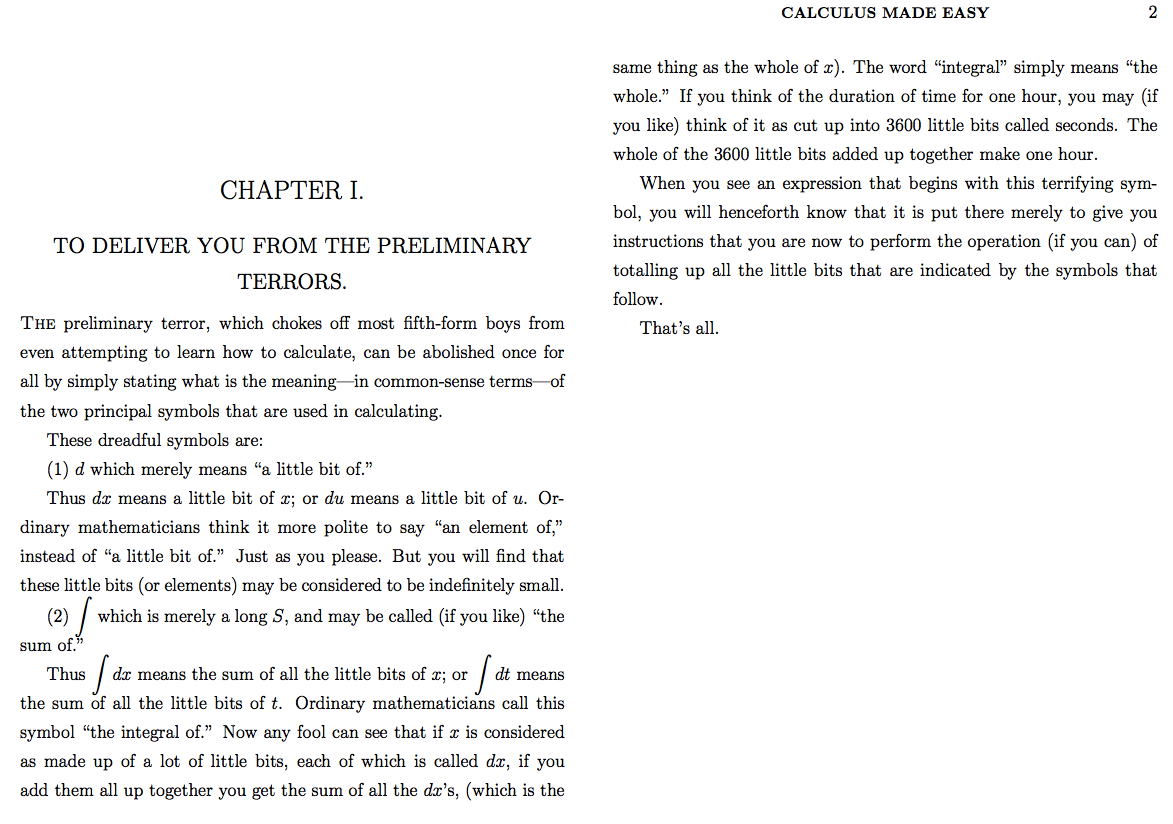# Possibly the easiest explanation of differentiation and Integration in Calculus

I have read a lot of books on Calculus but “Calculus Made Easy” by Silvanus P. Thompson stands out as possibly the easiest book to read on this topic. It totally lives up to its name. The first chapter opens up with an impressive introduction on the difference between dx∫.

d which merely means “a little bit of.”
Thus dx means a little bit of x; or du means a little bit of u.

∫ which is merely a long S, and may be called (if you like) “the sum of.”

Thusdx means the sum of all the little bits of x; or dt means the sum of all the little bits of t.

-“Calculus Made Easy” by Silvanus P. ThompsonSubscribe
Notify of